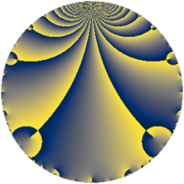# Properties

 Label 3822.2.a.rLevel $3822$ Weight $2$ Character orbit 3822.a Self dual yes Analytic conductor $30.519$ Analytic rank $0$ Dimension $1$ CM no Inner twists $1$

# Related objects

Show commands: Magma / PariGP / SageMath

## Newspace parameters

comment: Compute space of new eigenforms

[N,k,chi] = [3822,2,Mod(1,3822)]

mf = mfinit([N,k,chi],0)

lf = mfeigenbasis(mf)

from sage.modular.dirichlet import DirichletCharacter

H = DirichletGroup(3822, base_ring=CyclotomicField(2))

chi = DirichletCharacter(H, H._module([0, 0, 0]))

N = Newforms(chi, 2, names="a")

//Please install CHIMP (https://github.com/edgarcosta/CHIMP) if you want to run this code

chi := DirichletCharacter("3822.1");

S:= CuspForms(chi, 2);

N := Newforms(S);

 Level: $$N$$ $$=$$ $$3822 = 2 \cdot 3 \cdot 7^{2} \cdot 13$$ Weight: $$k$$ $$=$$ $$2$$ Character orbit: $$[\chi]$$ $$=$$ 3822.a (trivial)

## Newform invariants

comment: select newform

sage: f = N # Warning: the index may be different

gp: f = lf \\ Warning: the index may be different

 Self dual: yes Analytic conductor: $$30.5188236525$$ Analytic rank: $$0$$ Dimension: $$1$$ Coefficient field: $$\mathbb{Q}$$ Coefficient ring: $$\mathbb{Z}$$ Coefficient ring index: $$1$$ Twist minimal: yes Fricke sign: $$-1$$ Sato-Tate group: $\mathrm{SU}(2)$

## $q$-expansion

comment: q-expansion

sage: f.q_expansion() # note that sage often uses an isomorphic number field

gp: mfcoefs(f, 20)

 $$f(q)$$ $$=$$ $$q + q^{2} - q^{3} + q^{4} - 3 q^{5} - q^{6} + q^{8} + q^{9}+O(q^{10})$$ q + q^2 - q^3 + q^4 - 3 * q^5 - q^6 + q^8 + q^9 $$q + q^{2} - q^{3} + q^{4} - 3 q^{5} - q^{6} + q^{8} + q^{9} - 3 q^{10} - q^{11} - q^{12} + q^{13} + 3 q^{15} + q^{16} - 3 q^{17} + q^{18} + q^{19} - 3 q^{20} - q^{22} - q^{23} - q^{24} + 4 q^{25} + q^{26} - q^{27} + 5 q^{29} + 3 q^{30} - 6 q^{31} + q^{32} + q^{33} - 3 q^{34} + q^{36} - q^{37} + q^{38} - q^{39} - 3 q^{40} + 3 q^{43} - q^{44} - 3 q^{45} - q^{46} + 4 q^{47} - q^{48} + 4 q^{50} + 3 q^{51} + q^{52} - 6 q^{53} - q^{54} + 3 q^{55} - q^{57} + 5 q^{58} - 2 q^{59} + 3 q^{60} + q^{61} - 6 q^{62} + q^{64} - 3 q^{65} + q^{66} + 16 q^{67} - 3 q^{68} + q^{69} + 6 q^{71} + q^{72} + 5 q^{73} - q^{74} - 4 q^{75} + q^{76} - q^{78} + 12 q^{79} - 3 q^{80} + q^{81} - 6 q^{83} + 9 q^{85} + 3 q^{86} - 5 q^{87} - q^{88} - 3 q^{90} - q^{92} + 6 q^{93} + 4 q^{94} - 3 q^{95} - q^{96} + 18 q^{97} - q^{99}+O(q^{100})$$ q + q^2 - q^3 + q^4 - 3 * q^5 - q^6 + q^8 + q^9 - 3 * q^10 - q^11 - q^12 + q^13 + 3 * q^15 + q^16 - 3 * q^17 + q^18 + q^19 - 3 * q^20 - q^22 - q^23 - q^24 + 4 * q^25 + q^26 - q^27 + 5 * q^29 + 3 * q^30 - 6 * q^31 + q^32 + q^33 - 3 * q^34 + q^36 - q^37 + q^38 - q^39 - 3 * q^40 + 3 * q^43 - q^44 - 3 * q^45 - q^46 + 4 * q^47 - q^48 + 4 * q^50 + 3 * q^51 + q^52 - 6 * q^53 - q^54 + 3 * q^55 - q^57 + 5 * q^58 - 2 * q^59 + 3 * q^60 + q^61 - 6 * q^62 + q^64 - 3 * q^65 + q^66 + 16 * q^67 - 3 * q^68 + q^69 + 6 * q^71 + q^72 + 5 * q^73 - q^74 - 4 * q^75 + q^76 - q^78 + 12 * q^79 - 3 * q^80 + q^81 - 6 * q^83 + 9 * q^85 + 3 * q^86 - 5 * q^87 - q^88 - 3 * q^90 - q^92 + 6 * q^93 + 4 * q^94 - 3 * q^95 - q^96 + 18 * q^97 - q^99

## Embeddings

For each embedding $$\iota_m$$ of the coefficient field, the values $$\iota_m(a_n)$$ are shown below.

For more information on an embedded modular form you can click on its label.

comment: embeddings in the coefficient field

gp: mfembed(f)

Label $$\iota_m(\nu)$$ $$a_{2}$$ $$a_{3}$$ $$a_{4}$$ $$a_{5}$$ $$a_{6}$$ $$a_{7}$$ $$a_{8}$$ $$a_{9}$$ $$a_{10}$$
1.1
 0
1.00000 −1.00000 1.00000 −3.00000 −1.00000 0 1.00000 1.00000 −3.00000
 $$n$$: e.g. 2-40 or 990-1000 Significant digits: Format: Complex embeddings Normalized embeddings Satake parameters Satake angles

## Atkin-Lehner signs

$$p$$ Sign
$$2$$ $$-1$$
$$3$$ $$1$$
$$7$$ $$-1$$
$$13$$ $$-1$$

## Inner twists

This newform does not admit any (nontrivial) inner twists.

## Twists

By twisting character orbit
Char Parity Ord Mult Type Twist Min Dim
1.a even 1 1 trivial 3822.2.a.r 1
7.b odd 2 1 3822.2.a.bg yes 1

By twisted newform orbit
Twist Min Dim Char Parity Ord Mult Type
3822.2.a.r 1 1.a even 1 1 trivial
3822.2.a.bg yes 1 7.b odd 2 1

## Hecke kernels

This newform subspace can be constructed as the intersection of the kernels of the following linear operators acting on $$S_{2}^{\mathrm{new}}(\Gamma_0(3822))$$:

 $$T_{5} + 3$$ T5 + 3 $$T_{11} + 1$$ T11 + 1 $$T_{17} + 3$$ T17 + 3 $$T_{29} - 5$$ T29 - 5

## Hecke characteristic polynomials

$p$ $F_p(T)$
$2$ $$T - 1$$
$3$ $$T + 1$$
$5$ $$T + 3$$
$7$ $$T$$
$11$ $$T + 1$$
$13$ $$T - 1$$
$17$ $$T + 3$$
$19$ $$T - 1$$
$23$ $$T + 1$$
$29$ $$T - 5$$
$31$ $$T + 6$$
$37$ $$T + 1$$
$41$ $$T$$
$43$ $$T - 3$$
$47$ $$T - 4$$
$53$ $$T + 6$$
$59$ $$T + 2$$
$61$ $$T - 1$$
$67$ $$T - 16$$
$71$ $$T - 6$$
$73$ $$T - 5$$
$79$ $$T - 12$$
$83$ $$T + 6$$
$89$ $$T$$
$97$ $$T - 18$$